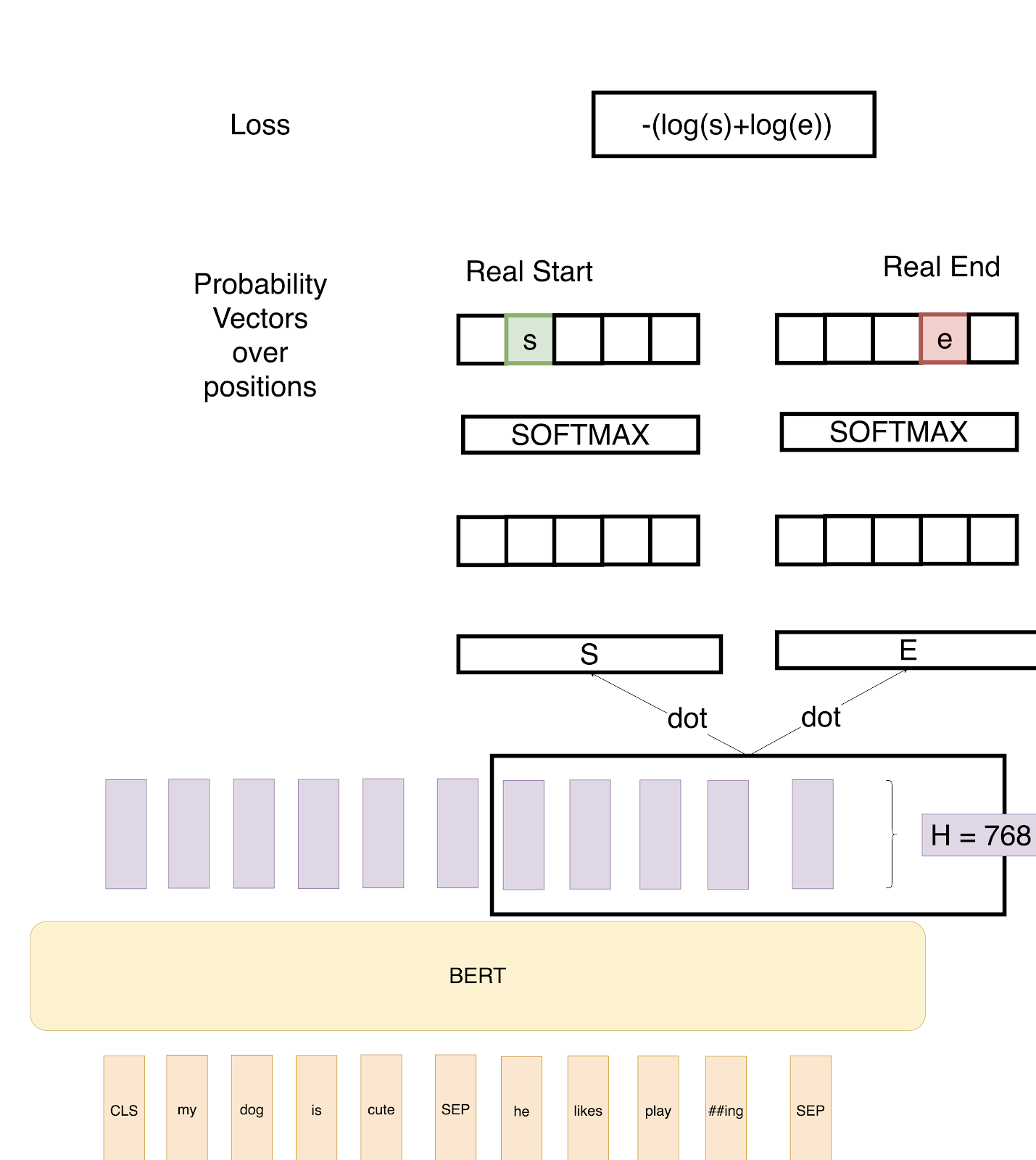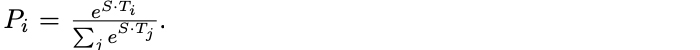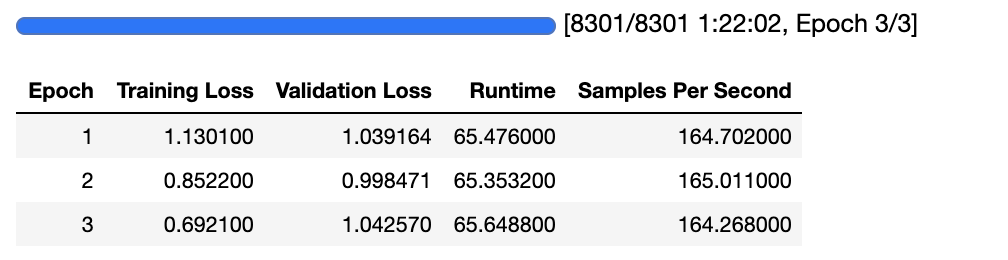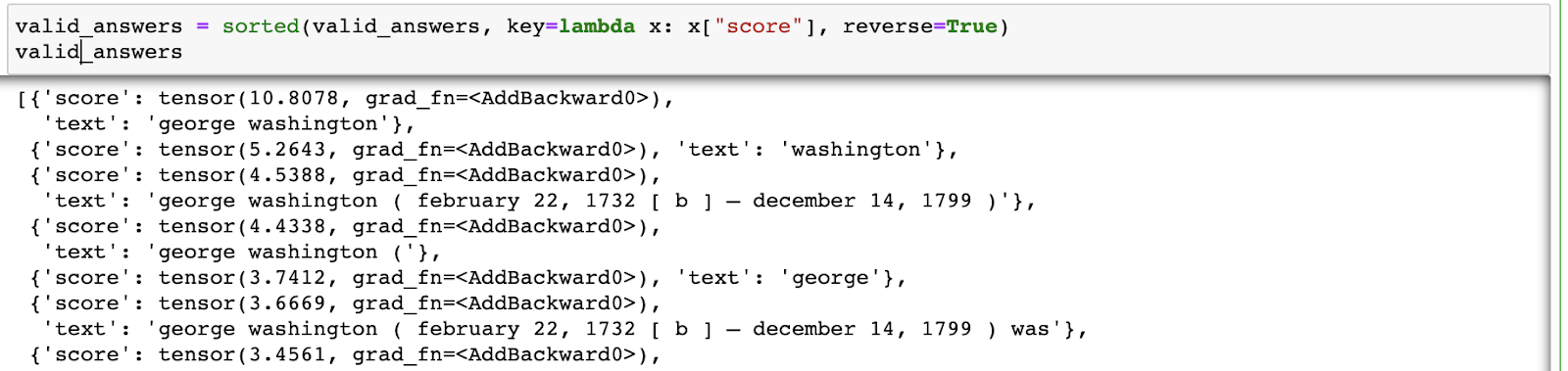Deep Learning

# Understanding BERT with Hugging Face

July 8, 2021### Using BERT and Hugging Face to Create a Question Answer Model

In a recent post on BERT, we discussed BERT transformers and how they work on a basic level. The article covers BERT architecture, training data, and training tasks.

However, we don’t really understand something before we implement it ourselves. So in this post, we will implement a Question Answering Neural Network using BERT and a Hugging Face Library.

In this task, we are given a question and a paragraph in which the answer lies to our BERT Architecture and the objective is to determine the start and end span for the answer in the paragraph.As explained in the previous post, in the above example we provide two inputs to the BERT architecture. The paragraph and the question are separated by the <SEP> token. The purple layers are the output of the BERT encoder.

We now define two vectors S and E (which will be learned during fine-tuning) both having shapes (1x768). We then take a dot product of these vectors with the second sentence’s output vectors from BERT, giving us some scores. We then apply Softmax over these scores to get probabilities. The training objective is the sum of the log-likelihoods of the correct start and end positions. Mathematically, for the Probability vector for Start positions:Where T_i is the word we are focusing on. An analogous formula is for End positions.

To predict a span, we get all the scores — S.T and E.T and get the best span as the span having the maximum Score, that is max(S.T_i + E.T_j) among all j≥i.

Interested in a deep learning workstation that can handle NLP training?

### How Do We Do This Using Hugging Face?

Hugging Face provides a pretty straightforward way to do this.

The output is:

Question: How many pretrained models are available in Transformers?

Question: What do Transformers provide?

Question: Transformers provide interoperability between which frameworks?

Answer: TensorFlow 2. 0 and PyTorch

So, here we just used the pretrained tokenizer and model on the SQuAD dataset provided by Hugging Face to get this done.

Once we have the model we just get the start and end probability scores and predict the span as the one that lies between the token that has the maximum start score and the token that has the maximum end score.

For example, if the start scores for paragraph are:

...
Transformers - 0.1
are - 0.2
general - 0.5
purpose - 0.1
architectures -0.01
(BERT - 0.001
...

And the end scores are:

...
Transformers - 0.01
are - 0.02
general - 0.05
purpose - 0.01
architectures -0.01
(BERT - 0.8
...

We will get the output as input_ids[answer_start:answer_end] where answer_start is the index of word general(one with max start score) and answer_end is index of (BERT(One with max end score). And the answer would be “general purpose architectures”.

### Fine-Tuning Our Own Model Using a Question-Answering Dataset

In most cases, we will want to train our own QA model on our own datasets. In this situation, we will start from the SQuAD dataset and the base BERT Model in the Hugging Face library to finetune it.

Let's look at how the SQuAD Dataset looks before we start fine tuning:

Context:Within computer systems, two of many security models capable of enforcing privilege separation are access control lists (ACLs) and capability-based security. Using ACLs to confine programs has been proven to be insecure in many situations, such as if the host computer can be tricked into indirectly allowing restricted file access, an issue known as the confused deputy problem. It has also been shown that the promise of ACLs of giving access to an object to only one person can never be guaranteed in practice. Both of these problems are resolved by capabilities. This does not mean practical flaws exist in all ACL-based systems, but only that the designers of certain utilities must take responsibility to ensure that they do not introduce flaws. [citation needed]

Question:The confused deputy problem and the problem of not guaranteeing only one person has access are resolved by what?

--------------------------------------------------------------------

Context:In recent years, the nightclubs on West 27th Street have succumbed to stiff competition from Manhattan's Meatpacking District about fifteen blocks south, and other venues in downtown Manhattan.

Question:How many blocks south of 27th Street is Manhattan's Meatpacking District?

--------------------------------------------------------------------

We can see each example containing the Context, Answer and the Start token for the Answer. We can use the script below to preprocess the data to the required format once we have the data in the above form. The script takes care of a lot of things amongst which the most important are the cases where the answer lies around max_length and calculating the span using the answer and the start token index.

Once we have data in the required format we can just fine tune our BERT base model from there.

```model_checkpoint = "bert-base-uncased"
tokenizer = AutoTokenizer.from_pretrained(model_checkpoint)tokenized_datasets = datasets.map(prepare_train_features, batched=True, remove_columns=datasets["train"].column_names)args = TrainingArguments(
evaluation_strategy = "epoch",
learning_rate=2e-5,
per_device_train_batch_size=16,
per_device_eval_batch_size=16,
num_train_epochs=3,
weight_decay=0.01,
)data_collator = default_data_collator
trainer = Trainer(
model,
args,
train_dataset=tokenized_datasets["train"],
eval_dataset=tokenized_datasets["validation"],
data_collator=data_collator,
tokenizer=tokenizer,
)trainer.train()Output from Fine Tuning BERT Base Model

Once we train our model we can use it as:

In this case also we take the index of max start scores and max end scores and predict the answer as the one that is between. If we want to get the exact implementation as provided in the BERT Paper we can tweak the above code a little and find out the indexes which maximize (start_score + end_score)Code Output from Model Training

### References

In this post, I covered how we can create a Question Answering Model from scratch using BERT. I hope it would have been useful both for understanding BERT as well as Hugging Face library.

If you want to look at other posts in this series check these out:

We will be writing more topics like this in the future, so let us know what you think about it. Should we write on heavily technical topics or aim more for beginner level? The comment section is your friend. Use it.

Have any questions?
Contact Exxact TodayDeep Learning

## Understanding BERT with Hugging Face

### Using BERT and Hugging Face to Create a Question Answer Model

In a recent post on BERT, we discussed BERT transformers and how they work on a basic level. The article covers BERT architecture, training data, and training tasks.

However, we don’t really understand something before we implement it ourselves. So in this post, we will implement a Question Answering Neural Network using BERT and a Hugging Face Library.

In this task, we are given a question and a paragraph in which the answer lies to our BERT Architecture and the objective is to determine the start and end span for the answer in the paragraph.As explained in the previous post, in the above example we provide two inputs to the BERT architecture. The paragraph and the question are separated by the <SEP> token. The purple layers are the output of the BERT encoder.

We now define two vectors S and E (which will be learned during fine-tuning) both having shapes (1x768). We then take a dot product of these vectors with the second sentence’s output vectors from BERT, giving us some scores. We then apply Softmax over these scores to get probabilities. The training objective is the sum of the log-likelihoods of the correct start and end positions. Mathematically, for the Probability vector for Start positions:Where T_i is the word we are focusing on. An analogous formula is for End positions.

To predict a span, we get all the scores — S.T and E.T and get the best span as the span having the maximum Score, that is max(S.T_i + E.T_j) among all j≥i.

Interested in a deep learning workstation that can handle NLP training?

### How Do We Do This Using Hugging Face?

Hugging Face provides a pretty straightforward way to do this.

The output is:

Question: How many pretrained models are available in Transformers?

Question: What do Transformers provide?

Question: Transformers provide interoperability between which frameworks?

Answer: TensorFlow 2. 0 and PyTorch

So, here we just used the pretrained tokenizer and model on the SQuAD dataset provided by Hugging Face to get this done.

Once we have the model we just get the start and end probability scores and predict the span as the one that lies between the token that has the maximum start score and the token that has the maximum end score.

For example, if the start scores for paragraph are:

...
Transformers - 0.1
are - 0.2
general - 0.5
purpose - 0.1
architectures -0.01
(BERT - 0.001
...

And the end scores are:

...
Transformers - 0.01
are - 0.02
general - 0.05
purpose - 0.01
architectures -0.01
(BERT - 0.8
...

We will get the output as input_ids[answer_start:answer_end] where answer_start is the index of word general(one with max start score) and answer_end is index of (BERT(One with max end score). And the answer would be “general purpose architectures”.

### Fine-Tuning Our Own Model Using a Question-Answering Dataset

In most cases, we will want to train our own QA model on our own datasets. In this situation, we will start from the SQuAD dataset and the base BERT Model in the Hugging Face library to finetune it.

Let's look at how the SQuAD Dataset looks before we start fine tuning:

Context:Within computer systems, two of many security models capable of enforcing privilege separation are access control lists (ACLs) and capability-based security. Using ACLs to confine programs has been proven to be insecure in many situations, such as if the host computer can be tricked into indirectly allowing restricted file access, an issue known as the confused deputy problem. It has also been shown that the promise of ACLs of giving access to an object to only one person can never be guaranteed in practice. Both of these problems are resolved by capabilities. This does not mean practical flaws exist in all ACL-based systems, but only that the designers of certain utilities must take responsibility to ensure that they do not introduce flaws. [citation needed]

Question:The confused deputy problem and the problem of not guaranteeing only one person has access are resolved by what?

--------------------------------------------------------------------

Context:In recent years, the nightclubs on West 27th Street have succumbed to stiff competition from Manhattan's Meatpacking District about fifteen blocks south, and other venues in downtown Manhattan.

Question:How many blocks south of 27th Street is Manhattan's Meatpacking District?

--------------------------------------------------------------------

We can see each example containing the Context, Answer and the Start token for the Answer. We can use the script below to preprocess the data to the required format once we have the data in the above form. The script takes care of a lot of things amongst which the most important are the cases where the answer lies around max_length and calculating the span using the answer and the start token index.

Once we have data in the required format we can just fine tune our BERT base model from there.

```model_checkpoint = "bert-base-uncased"
tokenizer = AutoTokenizer.from_pretrained(model_checkpoint)tokenized_datasets = datasets.map(prepare_train_features, batched=True, remove_columns=datasets["train"].column_names)args = TrainingArguments(
evaluation_strategy = "epoch",
learning_rate=2e-5,
per_device_train_batch_size=16,
per_device_eval_batch_size=16,
num_train_epochs=3,
weight_decay=0.01,
)data_collator = default_data_collator
trainer = Trainer(
model,
args,
train_dataset=tokenized_datasets["train"],
eval_dataset=tokenized_datasets["validation"],
data_collator=data_collator,
tokenizer=tokenizer,
)trainer.train()Output from Fine Tuning BERT Base Model

Once we train our model we can use it as:

In this case also we take the index of max start scores and max end scores and predict the answer as the one that is between. If we want to get the exact implementation as provided in the BERT Paper we can tweak the above code a little and find out the indexes which maximize (start_score + end_score)Code Output from Model Training

### References

In this post, I covered how we can create a Question Answering Model from scratch using BERT. I hope it would have been useful both for understanding BERT as well as Hugging Face library.

If you want to look at other posts in this series check these out:

We will be writing more topics like this in the future, so let us know what you think about it. Should we write on heavily technical topics or aim more for beginner level? The comment section is your friend. Use it.

Have any questions?
Contact Exxact Today